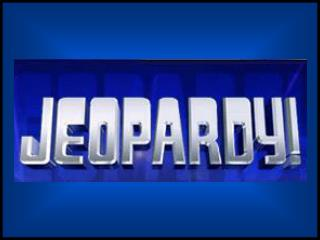DownloadDownload PresentationFinal Jeopardy

# Final Jeopardy

Download Presentation## Final Jeopardy

- - - - - - - - - - - - - - - - - - - - - - - - - - - E N D - - - - - - - - - - - - - - - - - - - - - - - - - - -
##### Presentation Transcript

1. Final Jeopardy Freezing Point Depression

2. Final Jeopardy 25g/58.5g/mol = .43 mol .43 mol/.250Kg = 1.7 m ∆T = 1.86oC/m x 1.7m x (2) = -6.3oC What is the freezing point of a solution made by dissolving 25 grams of NaCl in 250 mL of water?

3. \$200 Kinetic Molecular Theory This theory discusses the movement of molecules in their various states

4. \$400 Boyles Law The pressure and volume of a gas are inversely related

5. \$600 The atmosphere What is the SI unit for pressure?

6. \$800 The pressure will also increase Gay-Lussac would say as the temperature of a gas increases at constant volume

7. \$1000 Combined Gas Law PV = PV T T

8. \$200 Evaporation Process by which liquids change into a gas

9. \$400 Sublimation Phase change from a solid directly to a liquid

10. \$600 Gas In which state do the molecules have the greatest kinetic energy?

11. \$800 Temperature remains constant What happens to temperature during a phase change

12. \$1000 Triple Point Point at which all three states of matter can co-exist

13. \$200 Sand Sand, alloys, soda, which is not homogeneous?

14. \$400 Electrolyte A solution capable of conducting electricity

15. \$600 Compounds that are similar chemically dissolve other compounds that are alike What does “likes dissolve likes” refer to?

16. \$800 2 Molar 4 moles of a solute is dissolved in 2 Liters of solution, what is the Molarity

17. \$1000 PbCl2 Pb2+(aq) + 2 Cl-(aq) Write the equation for the dissolution of PbCl2(s) in water

18. \$200 supersaturated A solution in which no more solute can be dissolved

19. \$400 FP depression and BP elevation Name two colligative properties

20. \$600 Change in temp., molal constant, molality Using the equation ΔT = kf x m Explain the symbols

21. \$800 1 molal NaCl b/c it is an electrolyte and gives you two particles per mole Which would boil at a higher temperature and why? 1 molal glucose or 1 molal NaCl

22. \$1000 5 M, (.5 moles/.100L) Math Question: What is the molarity of 20 grams of NaOH dissolved in 100 mL of water

23. \$200 Base pH greater than 7

24. \$400 Acid [H+ ] > [OH-]

25. \$600 pH = - log [H+] What does pH equal

26. \$800 Sulfuric Acid What is the name of H2SO4

27. \$1000 1 x 10-7 Math Question: If pH = 7, then what is the [OH-]

28. \$200 Arrhenius Definition Bases produce OH- in water solutions

29. \$400 Whose definiton states that Acids are proton donors

30. \$600 8.7 What is pOH if pH = 5.3

31. \$800 pH = 10 [H+] = 1 x 10-10 What is the pH?

32. \$1000 pH = 9 [H+] = 1 x 10-9 so pH = 9 If [OH-] = 1 x 10-5 What is the pH?

33. \$400 Temperature Average kinetic energy of the molecules =

34. \$800 Specific Heat Amount of heat required to raise 1 gram of a substance 1oC

35. \$1200 Is equal to heat lost by the material In calorimetry experiments the heat gained by water

36. \$1600 J = 25g x 8 oC x 4.18 J/goC How much heat is required to raise 25 grams of water from 22oC to 30oC? Specific Heat of water = 4.18 J/goC

37. \$2000 Products should be higher than reactants Come to the board and draw a reaction that is endothermic

38. \$400 Low energy and high entropy In nature reactions tend to go towards what 2 directions?

39. \$800 Randomness or disorder What does entropy mean?

40. \$1200 Reaction mechanism A multistep process for a chemical reaction is called

41. \$1600 Activation energy The energy hump a reaction needs to overcome is called

42. \$2000 Temperature, surface area, catalyst, nature of reactants Name three (3) factors that affect reaction rate.

43. \$400 Rate of the Forward reaction equals the rate of the reverse reaction Define chemical equilibrium

44. \$800 Ka = [CH3COO-] [H3O+] [CH3COOH] Write the equilibrium expression for the following; CH3COOH(aq) + H2O(l) CH3COO-(aq) + H3O(aq)

45. \$1200 A large Ka value A strong acid will have what kind of value for it’s Ka ?

46. \$1600 Left N2(g) + 3 H2(g)  2 NH3(g) + 92 kJ If heat is added the reaction will shift?